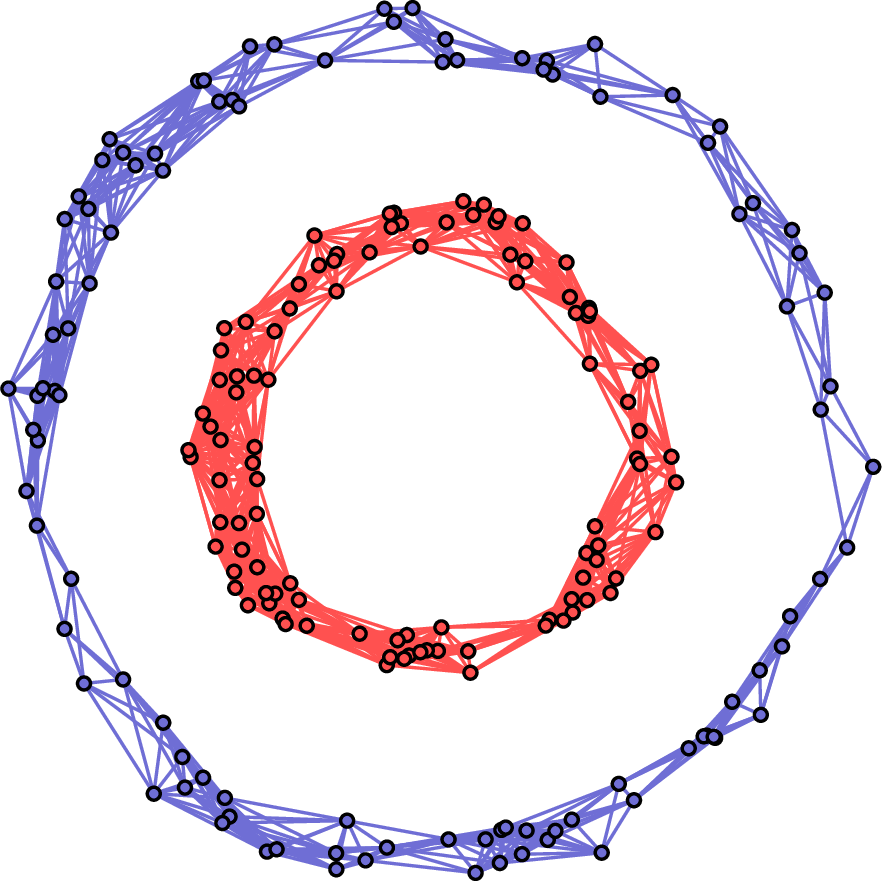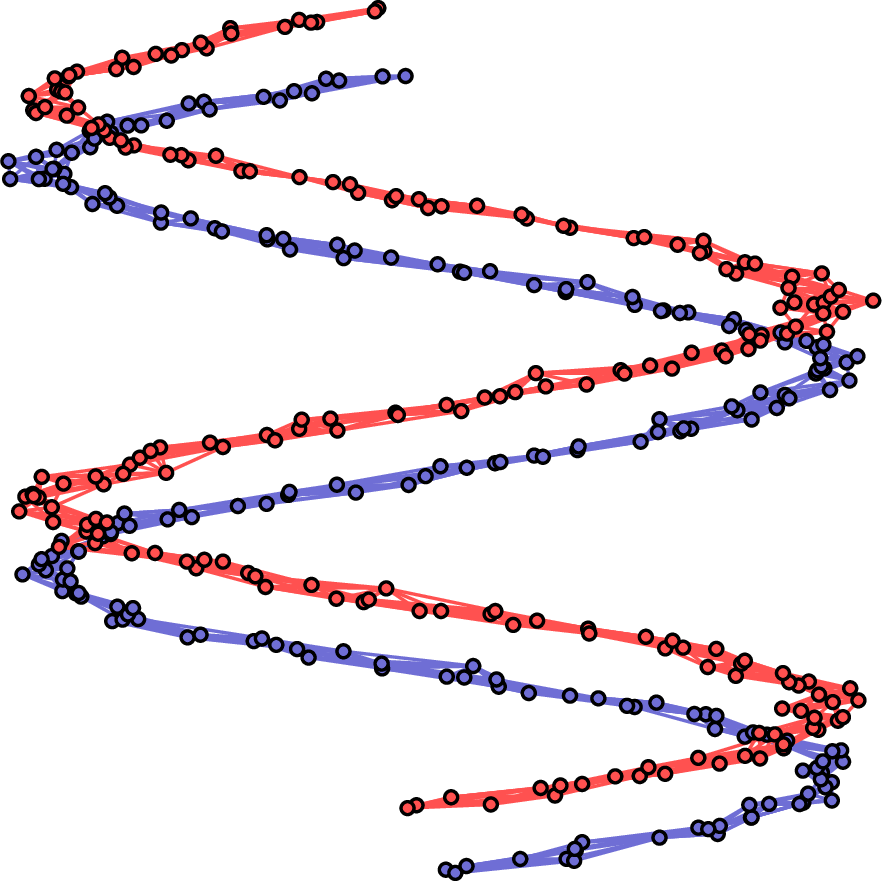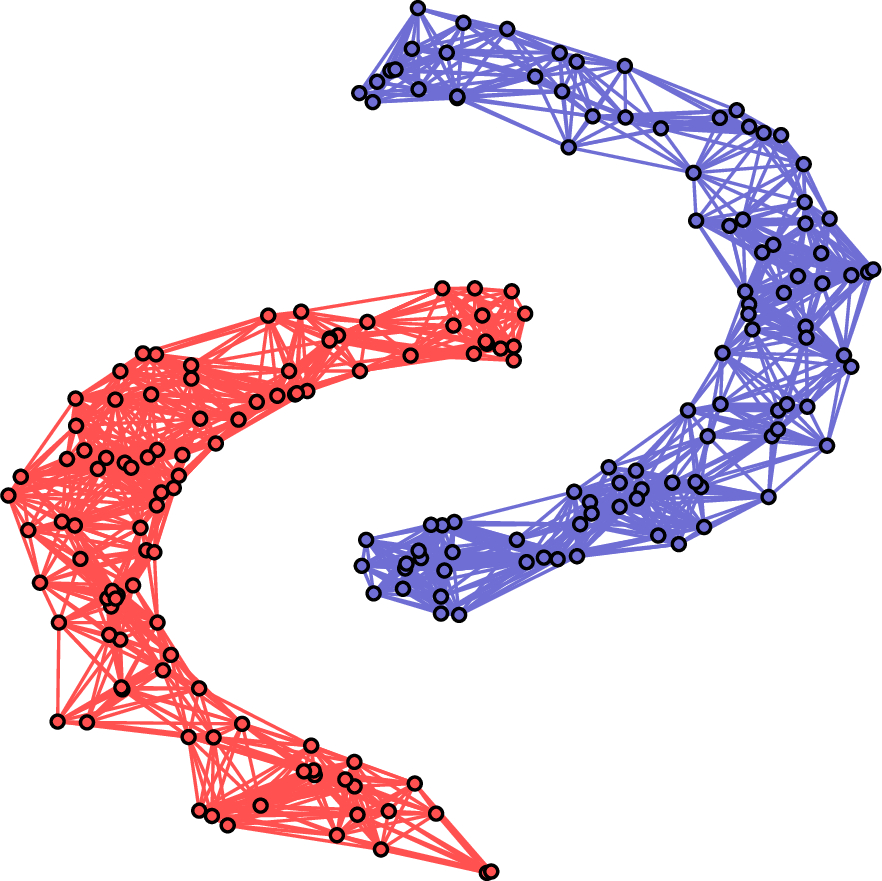# Package summary

spectralGraphTopology contains a collection of numerous efficient implementations of state-of-the-art algorithms designed to estimate graph matrices (Laplacian and Adjacency) from data, including:

# Installation

The development version of spectralGraphTopology can be installed from GitHub. While inside an R session, type:

> devtools::install_github("dppalomar/spectralGraphTopology")

Alternatively, the stable version of spectralGraphTopology can be installed from CRAN as follows: > install.packages("spectralGraphTopology")

For users of Windows, make sure to install the most current version of Rtools.

For users of macOS, make sure to install gfortran.

The help of the package can be accessed as follows: > help(package = 'spectralGraphTopology')

# Introduction

Graphs are one of the most popular mathematical structures that find applications in a myriad of scientific and engineering fields, such as finance, medical imaging, transportation, networks, and so on. In the era of big data and hyperconnectivity, graphs provides a way to model a vast diversity of phenomena, including customer preferences, brain activity, genetic structures, stock markets, just to name a few. Therefore, it is of utmost importance to be able to reliably estimate graph structures from noisy, often sparse, low-rank datasets.

A graph structure is defined by the Laplacian matrix of its graph, i.e., the Laplacian matrix contains the information about how nodes are connected among themselves. By definition a (combinatorial) Laplacian matrix is positive semi-definite, symmetric, and with sum of rows equal to zero. Therefore, one possible way to estimate a graph structure is through the estimation of its Laplacian matrix.

One common approach to estimate Laplacian matrices (without necessarily satisfying the zero row-sum property) would be via the inverse of the sample covariance matrix, which is an asymptotically unbiased and efficient estimator. In R, this estimator can be computed simply as S <- cov(X); solve(S), where X is the $$n \times p$$ data matrix, $$n$$ is the number of samples (or features) and $$p$$ is the number of nodes. This naive approach, although efficient when $$n$$ is orders of magnitude larger than $$p$$, poses way too many problems, e.g., if $$n \gtrsim p$$, then inverse of the sample covariance will likely be ill-conditioned, otherwise when $$n < p$$, the sample covariance matrix is low-rank, hence its inverse does not exist. In this case, one can resort to the pseudo-inverse of the sample covariance matrix, which in R can be computed using the MASS package as MASS::ginv(cov(X)). In practice, these naive techniques perform very poorly, even when $$n$$ is just a few orders of magnitude larger than $$p$$, which makes their use questionable or even impractical to be used in real-world problems.

Hence, the design of principled, efficient estimators for graph Laplacian matrices has attracted a substantial amount of attention from scientific communities including Machine Learning and Signal Processing.

In this package, we make available efficient implementations of state-of-the-art algorithms designed to estimate graph matrices (Laplacian and Adjancency) from data. We illustrate the practicality of some of these algorithms in clustering tasks.

# Connected graph formulation

Laplacian matrix estimation can be cast as a precision matrix estimation problem. Hence, the well-known graphical lasso algorithm can be used in this task. Graphical lasso was proposed in  where a maximum likelihood estimation (MLE) was formulated under a Gaussian Markov random field (GMRF) model including an $$\ell_1$$-norm penalty in order to induce sparsity on the solution. More precisely, the mathematical formulation for the graphical lasso problem can be expressed as follows: $\begin{array}{ll} \underset{\boldsymbol{\Theta} \succ \mathbf{0}}{\textsf{maximize}} & \log \det \boldsymbol{\Theta} - \mathrm{tr}(\mathbf{S}\boldsymbol{\Theta}) - \alpha \Vert \boldsymbol{\Theta}\Vert_{1, \text{off}}, \end{array}$ where $$\mathbf{S}$$ is the sample covariance matrix (or feature inner product matrix) and $$\alpha$$ is a hyperparameter that controls the amount of sparsity of the solution. This estimator has been efficiently implemented in the R package glasso.

The solution to the graphical lasso problem is a sparse precision matrix that may not satisfy the zero row-sum property of Laplacian matrices.

The authors in  considered to directly extend the graphical lasso framework so as to impose $$\boldsymbol{\Theta}$$ as a combinatorial graph Laplacian. Mathematically, the optimization problem becomes $\begin{equation} \begin{array}{ll} &\underset{\boldsymbol{\Theta}}{\textsf{maximize}} & \log \mathrm{gdet} \boldsymbol{\Theta} - \mathrm{tr}(\mathbf{S}\boldsymbol{\Theta}) - \alpha \Vert \boldsymbol{\Theta}\Vert_{1, \text{off}},\\ &\textsf{subject to} & \boldsymbol{\Theta} \in \mathcal{S}_{\mathcal{L}}, \end{array} \label{eq:cgl} \end{equation}$ where $$\mathrm{gdet} \boldsymbol{\Theta}$$ represents the generalized determinant, i.e., the product of the positive eigenvalues of $$\boldsymbol{\Theta}$$, and $$\mathcal{S}_{\mathcal{L}}$$ is the set of (combinatorial) Laplacian matrices, which may be written as $\begin{equation} \mathcal{S}_{\mathcal{L}} = \left\{\boldsymbol{\Theta} \in \mathbb{R}^{p \times p}: \boldsymbol{\Theta}\mathbf{1} = \mathbf{0}, \boldsymbol{\Theta}_{ij} = \boldsymbol{\Theta}_{ji} \le 0, \boldsymbol{\Theta} \succeq \mathbf{0}\right\}. \end{equation}$

It is worth noting that both graphical lasso and the combinatorial graph Laplacian (CGL) are convex problems that can be easily, albeit not efficiently, solved using disciplined convex programming frameworks such as CVX. A CVXR implementation of the above optimization problem can be coded as

learn_laplacian_matrix_cvx <- function(S, alpha = 0) {
p <- nrow(S)
ones <- rep(1, p)
zeros <- rep(0, p)
zeros_mat <- matrix(0, p, p)
J <- matrix(1/p, p, p)
H <- 2 * diag(p) - p * J
K <- S + alpha * H
Theta <- CVXR::Variable(p, p)
objective <- CVXR::matrix_trace(Theta %*% K) - CVXR::log_det(Theta + J)
constraints <- list(Theta == t(Theta),
Theta %*% ones == zeros,
Theta - CVXR::diag(CVXR::diag(Theta)) <= zeros_mat)
problem <- CVXR::Problem(CVXR::Minimize(objective), constraints)
result <- solve(problem)
return(list(Laplacian = result$getValue(Theta))) } In , the authors developed specialized optimization algorithms based on iterative block coordinate descent updates to solve the CGL problem. In a similar fashion, the authors in  proposed highly efficient algorithms to solve the CGL optimization problem using the frameworks Majorization-Minimization (MM) and Alternating Direction Method of Multipliers (ADMM). We set up a simple experiment to compare the performance of the four approaches: directly solving with CVXR, CGL , GLE-MM , and GLE-ADMM . We first build a grid graph with a total of 64 nodes using igraph::make_lattice and sample from it with increasing sample size ratios varying from 50 to 1000. We should expect to see a monotonically decreasing relative error curve as the sample size increases. Additionally, we should observe similar performances among the algorithms because after all the problem is convex. The snippet of code for this experiment is as follows: library(spectralGraphTopology) library(igraph) set.seed(42) # ratios between sample size and number of nodes ratios <- c(2, 5, 10, 50, 100, 250, 500, 1000) # number of nodes p <- 64 # generate a grid graph grid <- make_lattice(length = sqrt(p), dim = 2) # relative errors between the true Laplacian and the estimated ones re_mm <- rep(0, length(ratios)) re_admm <- rep(0, length(ratios)) re_cgl <- rep(0, length(ratios)) re_cvx <- rep(0, length(ratios)) for (k in c(1:length(ratios))) { # Randomly assign weights to the edges E(grid)$weight <- runif(gsize(grid), min = 1e-1, max = 3)
# Get the true Laplacian matrices
Ltrue <- as.matrix(laplacian_matrix(grid))
# Generate samples from the Laplacian matrix
X <- MASS::mvrnorm(ratios[k] * p, mu = rep(0, p), Sigma = MASS::ginv(Ltrue))
# Compute the sample covariance matrix
S <- cov(X)
# Estimate a graph from the samples using the MM method
graph_mm <- learn_laplacian_gle_mm(S = S, verbose = FALSE)
# Estimate a graph from the samples using the ADMM method
# Estimate a graph from the samples using the CGL method
graph_cgl <- learn_combinatorial_graph_laplacian(S = S, verbose = FALSE)
# Estimate a graph from the samples using CVX
graph_cvx <- learn_laplacian_matrix_cvx(S = S)
# record relative error between true and estimated Laplacians
re_mm[k] <- relative_error(Ltrue, graph_mm$Laplacian) re_admm[k] <- relative_error(Ltrue, graph_admm$Laplacian)
re_cgl[k] <- relative_error(Ltrue, graph_cgl$Laplacian) re_cvx[k] <- relative_error(Ltrue, graph_cvx$Laplacian)
}
colors <- c("#0B032D", "#843B62", "#E87118", "#40739E")
pch <- c(11, 7, 5, 6)
lty <- c(1:4)
legend <- c("MM", "ADMM", "CGL", "CVX")
xlab <- latex2exp::TeX("$\\mathit{n} / \\mathit{p}$")
par(bg = NA)
plot(c(1:length(ratios)), re_mm, ylim=c(min(re_admm) - 1e-3, max(re_cgl) + 1e-3), xlab = xlab,
ylab = "Relative Error", type = "b", lty=lty, pch=pch,
cex=.75, col = colors, xaxt = "n")
lines(c(1:length(ratios)), re_admm, type = "b", lty=lty, pch=pch,
cex=.75, col = colors, xaxt = "n")
lines(c(1:length(ratios)), re_cgl, type = "b", lty=lty, pch=pch,
cex=.75, col = colors, xaxt = "n")
lines(c(1:length(ratios)), re_cvx, type = "b", lty=lty, pch=pch, cex=.75, col = colors, xaxt = "n")
axis(side = 1, at = c(1:length(ratios)), labels = ratios)
legend("topright", legend=legend, col=colors, pch=pch, lty=lty, bty="n")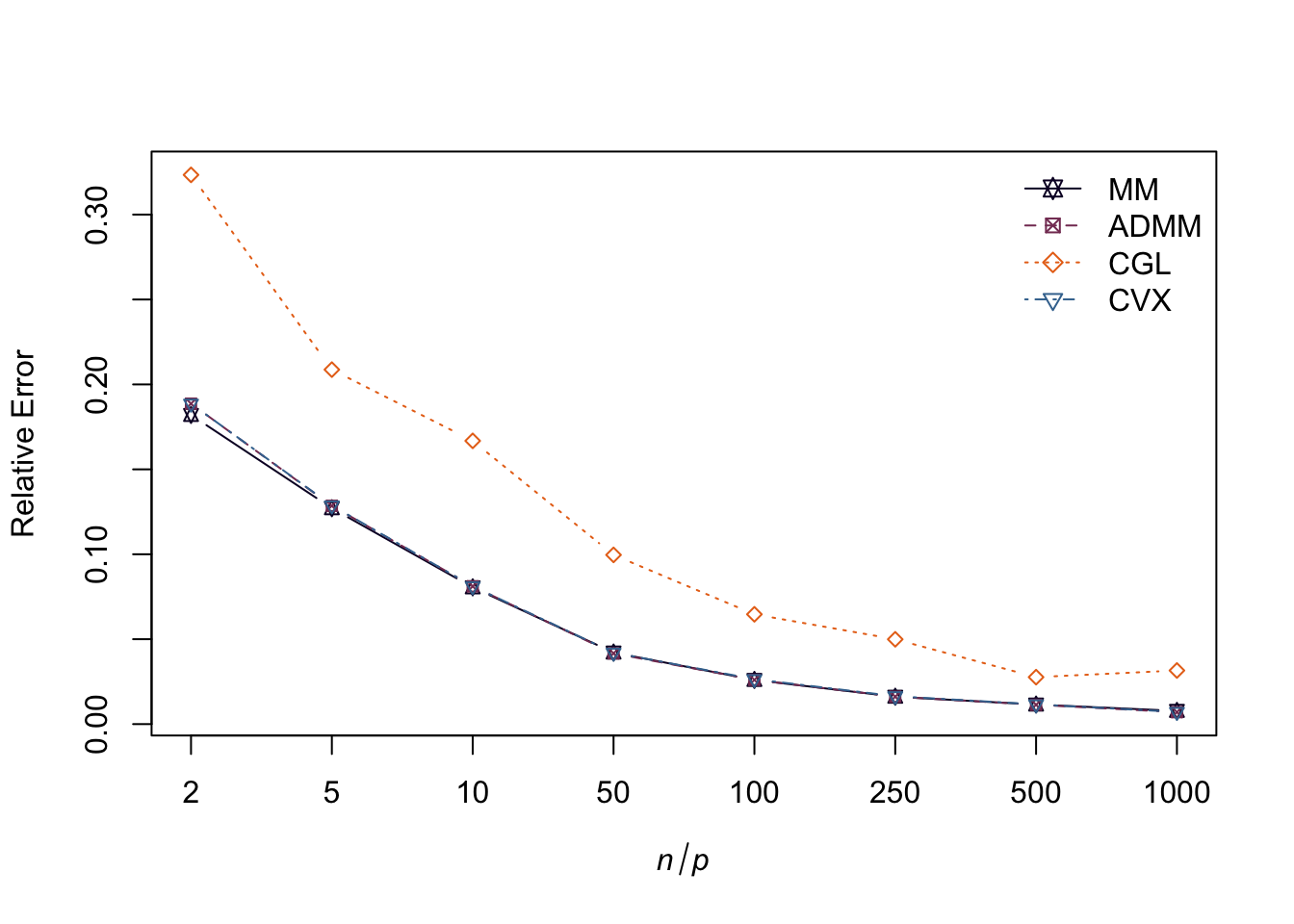Let’s also checkout the convergence curve of the objective function for each algorithm:

graph_mm <- learn_laplacian_gle_mm(S = S, record_objective = TRUE, verbose = FALSE)
graph_cgl <- learn_combinatorial_graph_laplacian(S = S, record_objective = TRUE, verbose = FALSE)
iter <- c(1:100)
plot(iter, graph_mm$obj_fun[iter], xlab = "number of iterations", ylab = "Objective function value", type = "b", lty=lty, pch=pch, cex=.75, col = colors) lines(iter, graph_admm$obj_fun[iter], type = "b", lty=lty, pch=pch,
cex=.75, col = colors)
lines(c(1:length(graph_cgl$obj_fun)), graph_cgl$obj_fun, type = "b", lty=lty, pch=pch,
cex=.75, col = colors)
legend("topright", legend=legend[1:3], col=colors[1:3], pch=pch[1:3], lty=lty[1:3], bty="n")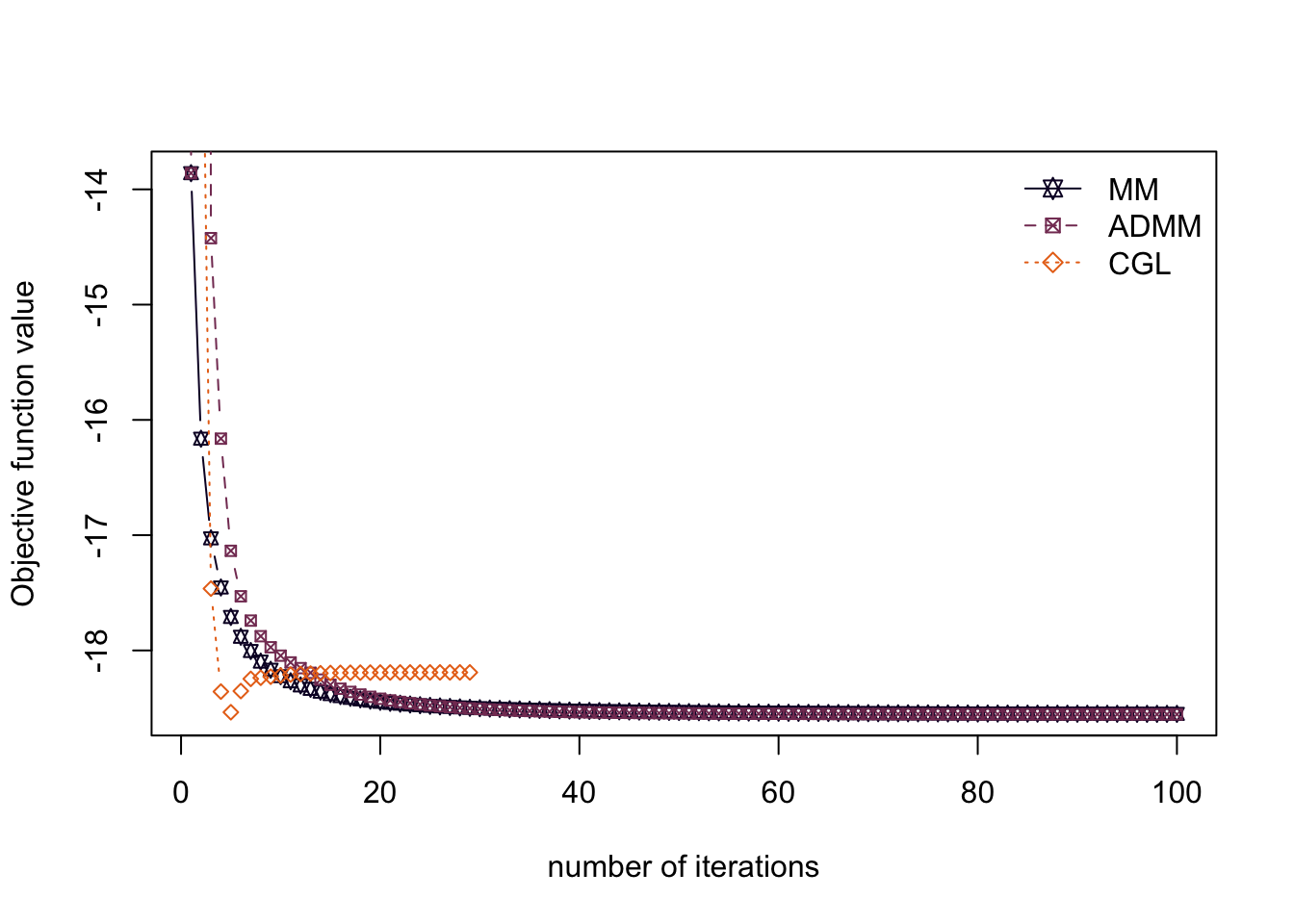Now, let’s compare the running time for each algorithm:

library(microbenchmark)
library(ggplot2)

op <- microbenchmark(
MM = learn_laplacian_gle_mm(S = S, verbose = FALSE),
CGL = learn_combinatorial_graph_laplacian(S = S, verbose = FALSE),
CVX = learn_laplacian_matrix_cvx(S = S), times = 10L)
autoplot(op)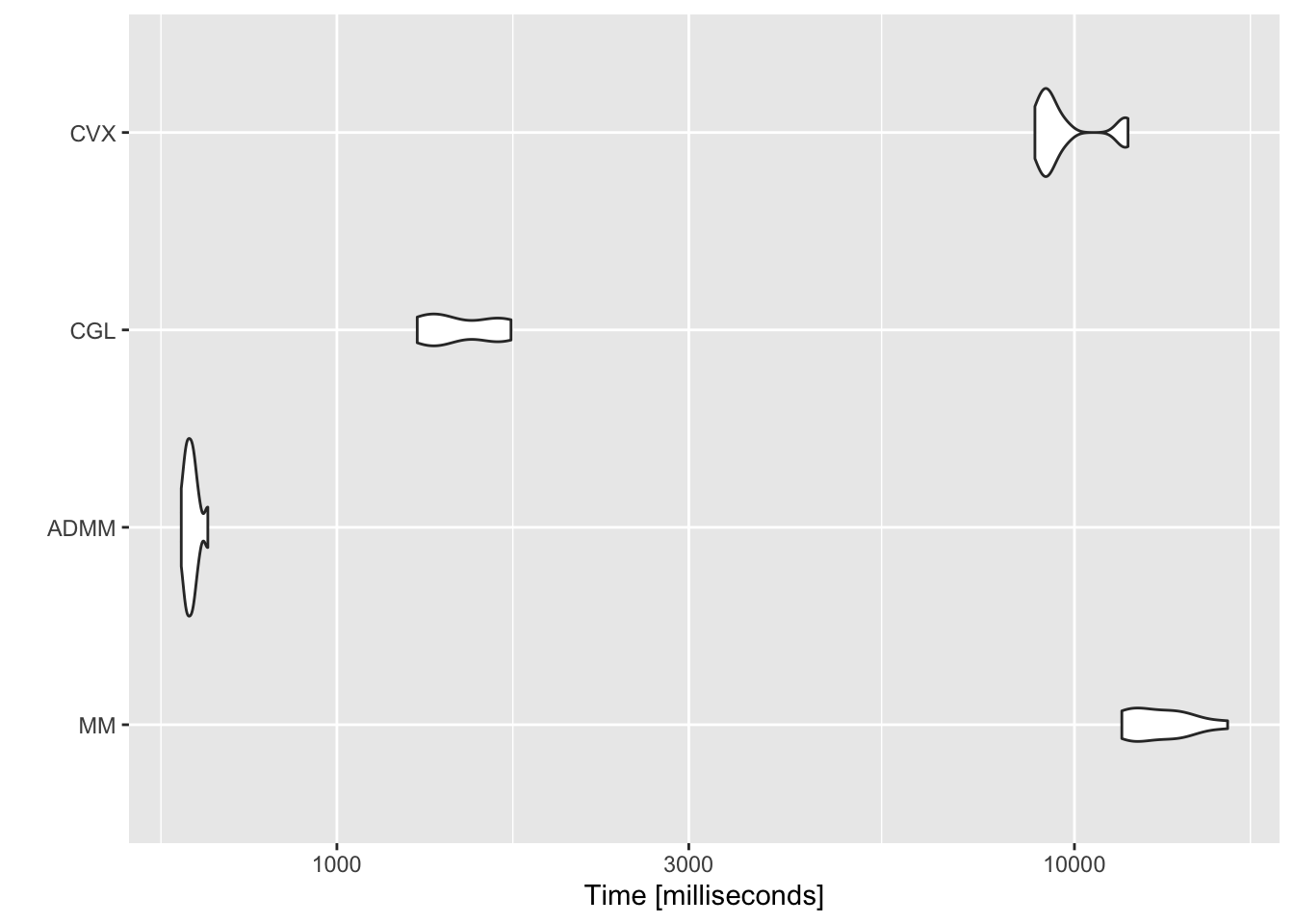In this toy example, as we can observe from the charts above, the methods MM, ADMM, and CVX are somewhat better than CGL when it comes to performance. In running time, CGL and ADMM seem to have a clear edge, while MM looks slow. However, the authors in  point out that the MM method should be more suitable for very sparse graphs. Therefore, we encourage users to try out different algorithms in their problems before drawing any conclusion.

# Introducing structural prior information

Although estimating a graph Laplacian matrix by solving formulation () has shown an extreme potential for success, this particular formulation only allows for the estimation of connected graphs. In many practical situations, however, graphs of more complex structures need to be estimated, such as k-component graphs, which are widely used in clustering tasks. Additionally, the structure of the graph might be known a priori, i.e., whether the graph is k-component, bipartite, k-component bipartite; and there should be a natural way of incorporating this prior knowledge into the optimization framework.

In this sense, in , we included spectral constraints into the regularized maximum likelihood framework as follows: $\begin{array}{ll} \underset{\boldsymbol{\Theta}}{\textsf{maximize}} & \log \mathrm{gdet} \boldsymbol{\Theta} - \mathrm{tr}(\mathbf{S}\boldsymbol{\Theta}) - \alpha \Vert \boldsymbol{\Theta}\Vert_{1}, \\ \textsf{subject to} & \boldsymbol{\Theta} \in \mathcal{S}_{\mathcal{L}}, \boldsymbol{\lambda}(\boldsymbol{\Theta}) \in \mathcal{S}_{\boldsymbol{\Lambda}}, \end{array}$

where $$\mathcal{S}_{\boldsymbol{\Lambda}}$$ is the set of vectors that constrains the eigenvalues of the Laplacian matrix. For example, for a $$k$$-component graph with $$p$$ nodes, $$\mathcal{S}_{\boldsymbol{\Lambda}} = \left\{\{\lambda_i\}_{i=1}^{p} | \lambda_1 = \lambda_2 = \cdots = \lambda_k = 0,\; 0 < \lambda_{k+1} \leq \lambda_{k+2} \leq \cdots \leq \lambda_{p} \right\}$$.

Now, realizing that for any $$\boldsymbol{\Theta} \in \mathcal{S}_{\mathcal{L}}$$, $$\boldsymbol{\Theta} = \mathcal{L}\mathbf{w}$$, where $$\mathcal{L} : \mathbb{R}^{p(p-1)/2} \rightarrow \boldsymbol{\Theta}$$ is a linear operator that maps a non-negative vector of edge weights $$\mathbf{w}$$ into a Laplacian matrix $$\boldsymbol{\Theta}$$, and that $$\boldsymbol{\Theta}$$ can be factorized as $$\boldsymbol{\Theta} = \mathbf{U}\boldsymbol{\Lambda}\mathbf{U}^\top$$, the original optimization problem may be approximated as follows: $\begin{array}{ll} \underset{\mathbf{w}, \boldsymbol{\lambda}, \mathbf{U}}{\textsf{minimize}} & - \log \textrm{gdet}\left({\sf Diag}(\boldsymbol{\lambda})\right) + \mathrm{tr}\left(\mathbf{S}\mathcal{L}\mathbf{w}\right) + \alpha \Vert\mathcal{L}\mathbf{w}\Vert_{1} + \frac{\beta}{2}\big\|\mathcal{L}\mathbf{w} - \mathbf{U}{\sf Diag}(\boldsymbol{\lambda})\mathbf{U}^{T}\big\|^{2}_{F},\\ \textsf{subject to} & \mathbf{w} \geq 0, \boldsymbol{\lambda} \in \mathcal{S}_{\boldsymbol{\Lambda}},~\text{and}~ \mathbf{U}^{T}\mathbf{U} = \mathbf{I}. \end{array}$

To solve this optimization problem, we employ a block majorization-minimization framework that updates each of the variables ($$\mathbf{w}, \boldsymbol{\lambda}, \mathbf{U}$$) at once while fixing the remaning ones. For the mathematical details of the solution, including a convergence proof, please refer to our paper .

In order to learn bipartite graphs, we take advantage of the fact that the eigenvalues of the adjacency matrix of graph are symmetric around 0, and we formulate the following optimization problem: $\begin{array}{ll} \underset{{\mathbf{w}},{\boldsymbol{\psi}},{\mathbf{V}}}{\textsf{minimize}} & \begin{array}{c} - \log \det (\mathcal{L} \mathbf{w}+\frac{1}{p}\mathbf{11}^{T})+\text{tr}({\mathbf{S}\mathcal{L} \mathbf{w}})+ \alpha \Vert\mathcal{L}\mathbf{w}\Vert_{1}+ \frac{\gamma}{2}\Vert \mathcal{A} \mathbf{w}-\mathbf{V} {\sf Diag}(\boldsymbol{\psi}) \mathbf{V}^T \Vert_F^2, \end{array}\\ \text{subject to} & \begin{array}[t]{l} \mathbf{w} \geq 0, \ \boldsymbol{\psi} \in \mathcal{S}_{\boldsymbol{\psi}}, \ \text{and} \ \mathbf{V}^T\mathbf{V}=\mathbf{I}, \end{array} \end{array}$ where $$\mathcal{A}$$ is a linear operator that maps a non-negative vector of edge weights $$\mathbf{w}$$ into an adjacency matrix.

In a similar fashion, we construct the optimization problem to estimate a $$k$$-component bipartite graph by combining the constraints related to the Laplacian and adjacency matrices.

# Learning k-component, bipartite, and k-component bipartite graphs

The spectralGraphTopology package provides three main functions to estimate k-component, bipartite, and k-component bipartite graphs, respectively: learn_k_component_graph, learn_bipartite_graph, and learn_bipartite_k_component_graph. In the next subsections, we will check out how to apply those functions in synthetic datasets.

## Clustering via k-component graphs

The next snippet of code shows how to learn the clusters of a set of points distributed on the two dimensional Euclidean space:

library(spectralGraphTopology)
library(clusterSim)
library(igraph)
set.seed(42)

# generate the graph and the data from the graph
n <- 100  # number of nodes per cluster
circles3 <- shapes.circles3(n)  # generate datapoints
k <- 3  # number of components
S <- crossprod(t(circles3$data)) # compute sample correlation matrix # estimate the graph graph <- learn_k_component_graph(S, k = k, beta = 1, verbose = FALSE, fix_beta = FALSE, abstol = 1e-3) # plots # build network net <- graph_from_adjacency_matrix(graph$Adjacency, mode = "undirected", weighted = TRUE)
# colorify nodes and edges
colors <- c("#706FD3", "#FF5252", "#33D9B2")
V(net)$cluster <- circles3$clusters
E(net)$color <- apply(as.data.frame(get.edgelist(net)), 1, function(x) ifelse(V(net)$cluster[x] == V(net)$cluster[x], colors[V(net)$cluster[x]], '#000000'))
V(net)$color <- colors[circles3$clusters]
par(bg = NA)
plot(net, layout = circles3\$data, vertex.label = NA, vertex.size = 3)
title("Estimated graph on the three circles dataset")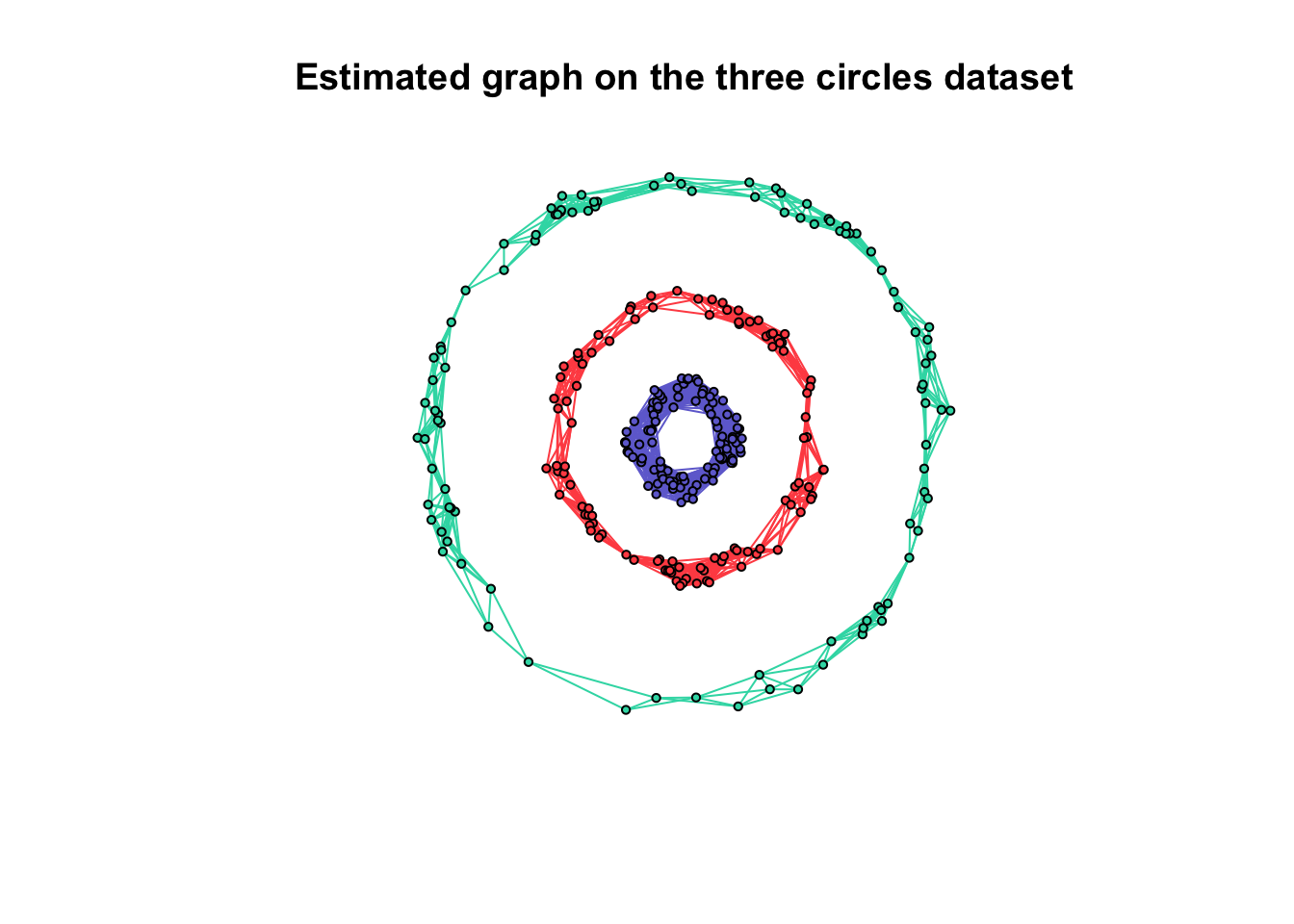Similar structures may be inferred as well. The plots below depict the results of applying learn_k_component_graph() on a variaety of spatially distributed points.